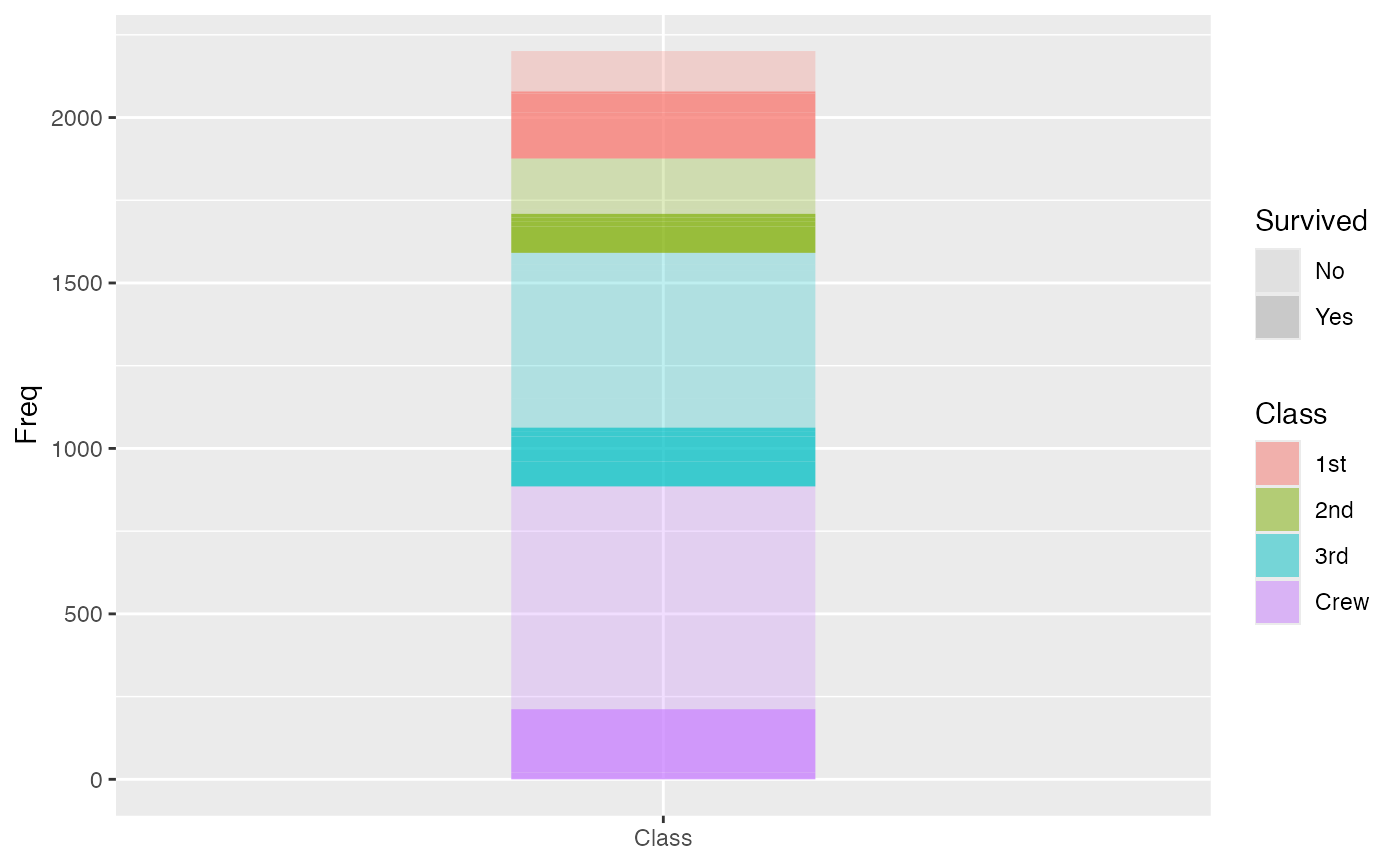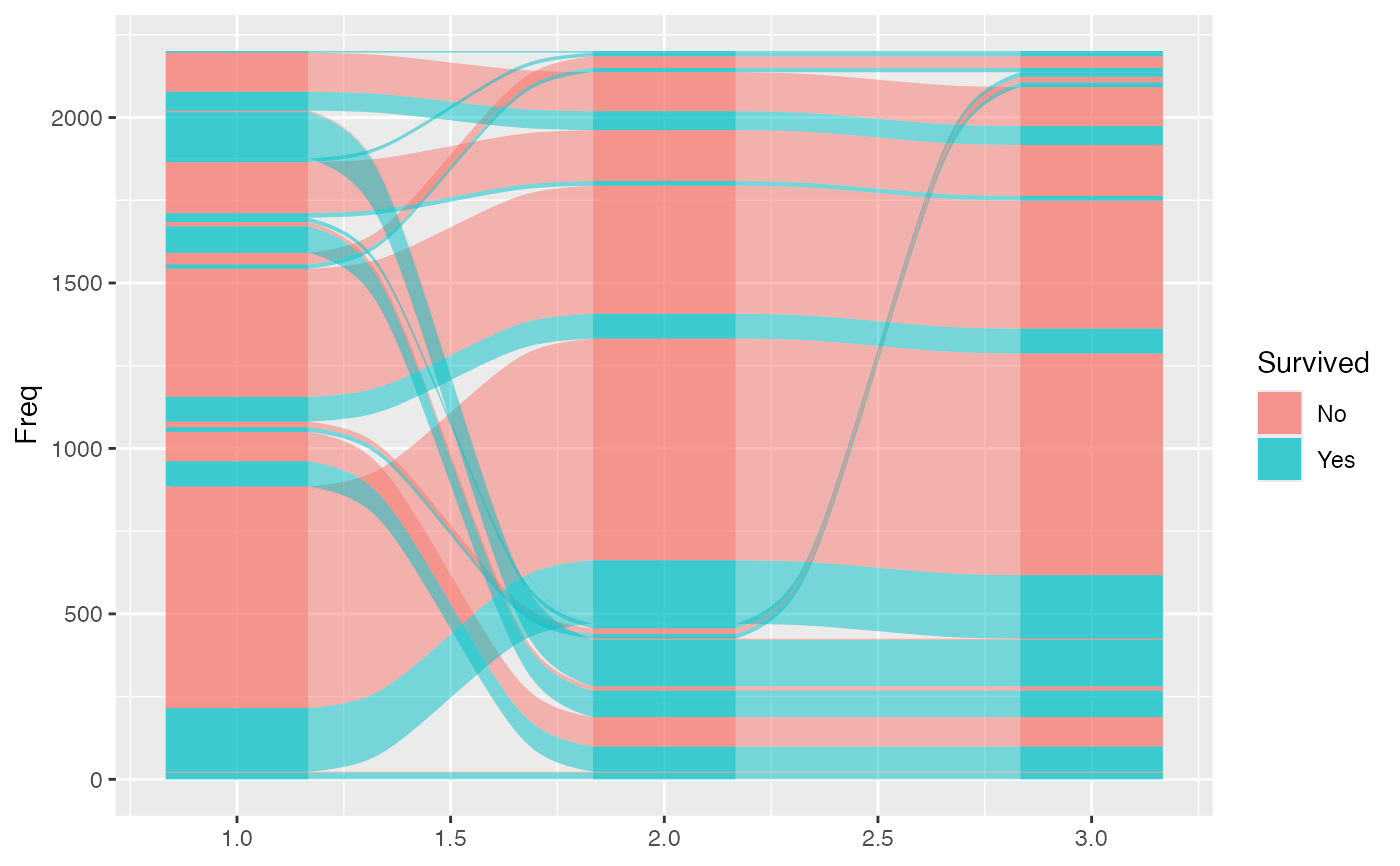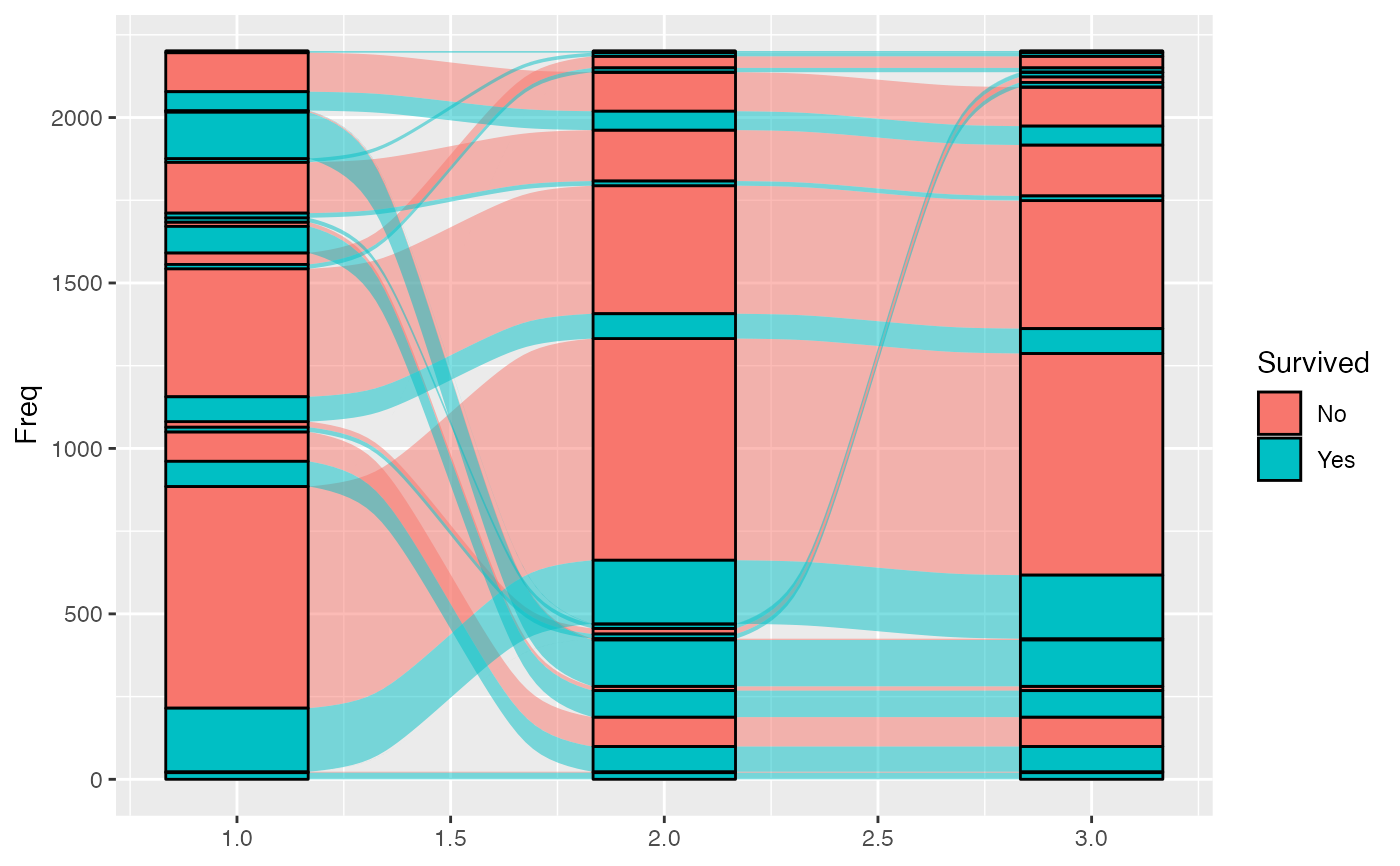geom_alluvium receives a dataset of the horizontal (x) and vertical (y, ymin, ymax) positions of the lodes of an alluvial plot, the intersections of the alluvia with the strata. It plots rectangles for these lodes of a provided width.

geom_lode(
mapping = NULL,
data = NULL,
stat = "alluvium",
position = "identity",
width = 1/3,
na.rm = FALSE,
show.legend = NA,
inherit.aes = TRUE,
...
)

## Arguments

mapping

Set of aesthetic mappings created by aes(). If specified and inherit.aes = TRUE (the default), it is combined with the default mapping at the top level of the plot. You must supply mapping if there is no plot mapping.

data

The data to be displayed in this layer. There are three options:

If NULL, the default, the data is inherited from the plot data as specified in the call to ggplot().

A data.frame, or other object, will override the plot data. All objects will be fortified to produce a data frame. See fortify() for which variables will be created.

A function will be called with a single argument, the plot data. The return value must be a data.frame, and will be used as the layer data. A function can be created from a formula (e.g. ~ head(.x, 10)).

stat

The statistical transformation to use on the data; override the default.

position

Position adjustment, either as a string naming the adjustment (e.g. "jitter" to use position_jitter), or the result of a call to a position adjustment function. Use the latter if you need to change the settings of the adjustment.

width

Numeric; the width of each stratum, as a proportion of the distance between axes. Defaults to 1/3.

na.rm

Logical: if FALSE, the default, NA lodes are not included; if TRUE, NA lodes constitute a separate category, plotted in grey (regardless of the color scheme).

show.legend

logical. Should this layer be included in the legends? NA, the default, includes if any aesthetics are mapped. FALSE never includes, and TRUE always includes. It can also be a named logical vector to finely select the aesthetics to display.

inherit.aes

If FALSE, overrides the default aesthetics, rather than combining with them. This is most useful for helper functions that define both data and aesthetics and shouldn't inherit behaviour from the default plot specification, e.g. borders().

...

Additional arguments passed to ggplot2::layer().

## Aesthetics

geom_alluvium, geom_flow, geom_lode, and geom_stratum understand the following aesthetics (required aesthetics are in bold):

• x

• y

• ymin

• ymax

• alpha

• colour

• fill

• linetype

• size

• group

group is used internally; arguments are ignored.

## Defunct parameters

The previously defunct parameters axis_width and ribbon_bend have been discontinued. Use width and knot.pos instead.

ggplot2::layer() for additional arguments and stat_alluvium() and stat_stratum() for the corresponding stats.

Other alluvial geom layers: geom_alluvium(), geom_flow(), geom_stratum()

## Examples

# one axis
ggplot(as.data.frame(Titanic),
aes(y = Freq,
axis = Class)) +
geom_lode(aes(fill = Class, alpha = Survived)) +
scale_x_discrete(limits = c("Class")) +
scale_alpha_manual(values = c(.25, .75))gg <- ggplot(as.data.frame(Titanic),
aes(y = Freq,
axis1 = Class, axis2 = Sex, axis3 = Age,
fill = Survived))
# alluvia and lodes
gg + geom_alluvium() + geom_lode()# lodes as strata
gg + geom_alluvium() +
geom_stratum(stat = "alluvium")